# Abstracts

In order to support the theoretical basis and contribute to the improvement of educational capability issues relating to irrigation systems design, this point of view presents an alternative deduction of the variance of the discharge as a bidimensional and independent random variable. Then a subsequent brief application of an existing model is applied for statistical design of laterals in micro-irrigation. The better manufacturing precision of emitters allows lengthening a lateral for a given soil slope, although this does not necessarily mean that the statistical uniformity throughout the lateral will be more homogenous.

error theory; statistical approach; manufacturing variation

Visando a reforçar as bases teóricas e contribuir com a melhoria da capacitação educacional em assuntos relacionados a dimensionamento de sistemas de irrigação, o presente ponto de vista revela uma dedução alternativa para a variância da vazão dos emissores, variável aleatória independente bidimensional. Posteriormente apresenta breve aplicação do modelo aceito para dimensionamento de linhas laterais em sistemas de microirrigação, de acordo com a abordagem estatística. A melhor precisão na fabricação de emssores permite, para uma dada inclinação de terreno, dimensionar laterais mais longas, o que não significa necessariamente que uniformidade de emissão dessas laterais será projetada como mais homogênea.

teoria dos erros; dimensionamento estatístico; variação de fabricação

POINT OF VIEW

Theoretical basis and significance of the variance of discharge as a bidimensional variable for the design of lateral lines of micro-irrigation

Bases teóricas e importância da variância da vazão como variável bidimensional no dimensionamento de linhas laterais em microirrigação

Euro Roberto DetominiI; Brendan PowerII; José Antônio FrizzoneIII, * * Corresponding author < frizzone@esalq.usp.br>

IUSP/ESALQ - Programa de Pós-Graduação em Engenharia Agrícola, C.P. 09 - 13418-900 - Piracicaba, SP - Brasil

IIDept. of Primary Industries & Fisheries, PO BOX 103 - 4350 - Toowoomba - QLD, Australia

IIIUSP/ESALQ - Depto. Engenharia Rural, C.P. 09 - 13418-900 - Piracicaba, SP - Brasil

ABSTRACT

In order to support the theoretical basis and contribute to the improvement of educational capability issues relating to irrigation systems design, this point of view presents an alternative deduction of the variance of the discharge as a bidimensional and independent random variable. Then a subsequent brief application of an existing model is applied for statistical design of laterals in micro-irrigation. The better manufacturing precision of emitters allows lengthening a lateral for a given soil slope, although this does not necessarily mean that the statistical uniformity throughout the lateral will be more homogenous.

Key words: error theory, statistical approach, manufacturing variation

RESUMO

Visando a reforçar as bases teóricas e contribuir com a melhoria da capacitação educacional em assuntos relacionados a dimensionamento de sistemas de irrigação, o presente ponto de vista revela uma dedução alternativa para a variância da vazão dos emissores, variável aleatória independente bidimensional. Posteriormente apresenta breve aplicação do modelo aceito para dimensionamento de linhas laterais em sistemas de microirrigação, de acordo com a abordagem estatística. A melhor precisão na fabricação de emssores permite, para uma dada inclinação de terreno, dimensionar laterais mais longas, o que não significa necessariamente que uniformidade de emissão dessas laterais será projetada como mais homogênea.

Palavras-chave: teoria dos erros, dimensionamento estatístico, variação de fabricação

INTRODUCTION

In micro-irrigation systems, the longer the lateral pipelines are implemented in a submain unit,,the less is the final cost of the designed project, collaborating with the overall profitability of the system. In practice, the hydraulic and the statistical approaches may be used to optimise the lateral length of the pipelines while maintaining the desirable uniformity of applied water. Considering the latter, the coefficient of variation of the pressure head (CVHp) term appears in the model due to the concept of discharge variance, where the source-point discharge is assumed to be susceptible to oscillations in both pressure head (H) and emitter coefficient (K), which is inherent to the existing variability of emitters due to manufacturing processes. Specifically, discharge variation values are assumed to follow a bivariate normal distribution without correlation between independent variables and hence these oscillations need to be contemplated when designing laterals. Small differences between supposedly identical emitters may result in significant discharge variations.

The manufacturer's coefficient of emitter variation is a measure of the variability of the discharge of a random sample of a given make, model and size of the emitter as produced by the manufacturer, evaluted any field operation or ageing has taken place (ASAE, 1996). It reflects the degree of precision with which the emitters are made by the manufacturer. With respect to average source-point discharge, one can use Taylor's series todeduct the variance with some degree of difficulty or alternatively and easily via error theory. In order to support the theoretical basis and contribute to the improvement of educational capability issues relating to irrigation design, this work uses a simple algebraic manipulation used in engineering and thereby presents an alternative deduction of the variance of discharge as a bidimensional variable and a brief application of the Anyoji & Wu (1987) model to statistically design laterals in micro-irrigation.

MODEL DESCRIPTION AND ALTERNATIVE DEDUCTION

To describe the emitter discharge (q, L h1), Keller & Bliesner (1990) suggest the widely used power equation expressed as a function of pressure head (H, m):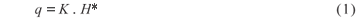The variance of discharge (δ q2) throughout a lateral pipeline is given by: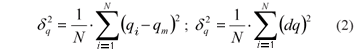in which qi is the discharge of the ith emitter; qm is the mean discharge; and dq is the deviation of discharge values (continuous variable); and N is the number of emitters along the lateral.

Likewise, the variances of the independent variables are:in which dq, dK and dH are the deviations of discharge, emitter constant and pressure head, respectively.

The coefficient of correlation (r) between the random variables K and H measures the magnitude and direction in which they linearly stretch together ahead (Moore, 1995; p.111), presented as: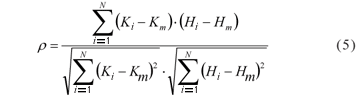Rearranging eq. (5) in a convenient manner we have:According to the error theory, the total derivative of eq. (1) corresponds to the total error implied when it is used. Particularly for q, this may be described by the chain rule:where:Whereas the higher-order partial differentiations are given by:Substituting eq. (7) into eq. (2):Expanding eq. (12) by taking the binomial quadratic expansion: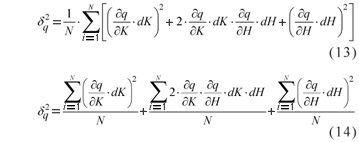All terms involving partial differentiation on the above equation are possible to be taken out of the operator sum because they do not contemplate the ith indices. This might be verified by rewriting eq. (8) and eq. (9). Thus:Substituting eq. (6) into eq. (15) we have: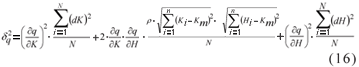Assuming that the variables K and H are independent of each other, especially when the system pressure is low and the emitter material is rigid enough against deformation, r is zero and the second term of second member vanishes in the equation above. Hence, eq. (16) reduces to:Substituting equations (3), (4), (8) and (9) into the equation above, we obtain:Provided that the coefficients of variation are considered, this highlights that:Substituting eq. (20) and eq. (21) into eq. (18):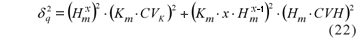Reorganising the equation above in a convenient way:Equation (23) is going to be utilised in the coming steps, and it represents the variance of a point-source of the discharge as a bidimensional variable, that depends upon both emitter coefficient (K) and pressure head (H), if clogging and temperature effects on emitter discharge are neglected (i.e. design stage).

APLICABILITY OF VARIANCE OF THE BIDIMENSIONAL DISCHARGE

The expectation [E(q)] of q utilising a Taylor's series expansion is:When all events have the same probability of occurrence, the expectation (or expected value) equals the arithmetic average, but the second term of second member stands for the variation of discharge due to the effect of the variation of both K and H. The expectation of q can also be called qm.

Substituting equations (10), (11) and (21), into 24 becomes: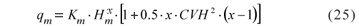Substituting both eq. (25) and eq. (23) into eq. (19), the latter becomes:As made in this presentation for the expectation of q [see eq. (24)], Anyoji and Wu (1987) have demonstrated eq. (26) by using the approach of Lindley (1965) about Taylor's Series to estimate the variance of a variable that is function of two independent variables, therefore, differently from the herein presented approach, based on error theory and fully deduced for the variance. The approach of Lindley (1965) is certainly and fully valid but it is more complex to deduce the variance than error theory, whereas the closed equation to deduce the expectation through Taylor's Series can be achieved in an easier way.

The variable CVK is often referred as CVf. Additionally, CVH might be called as CVHp (coefficient of variation of pressure on project) at the design stage, most likely to be different from the CVH of the working irrigation system. Considering this and conveniently rearranging the equation 26, we finally have the expression that, indeed, is the first step to statistically design laterals in irrigation systems:Since CVq is a user-defined value according to a uniformity criteria, generally not more than 0.07 (or 7%), indicating an emitter flow variation of 20% (Wu, 1997). The attributes CVf and x are known elements from manufacturers, whereas CVHp becomes the variable of interest. As it is not possible to explicitly derive CVHp, it must be found through any iterative method (i.e. Newton-Raphson, Bisection or Secant).

Even though Frizzone et al. (1998) have utilised eq. (26) for their calculations, which is basically the same as the one developed by Anyoji & Wu (1987) and the rearranged eq. (27), the latter is mathematically more suitable than the former in terms of programming, because it expresses a typical algebraic equation to be solved in zero [i.e. f(CVHp) = 0]. Burden & Faiures (2004) provide more details about the advantages and limitations of some of the main iterative methods in terms of facility and speed of convergence. As far as we have found, such value might be easily obtained by the root-finding algorithm of Microsoft Excel® (Tools > Goal Seek...), with no difficulty of convergence.

If the second term inside the brackets of the denominator of eq. (26) is small, as when x tends to 0.5 (i.e. working in full turbulence), the expression in the brackets [1 + 0.5 · x · CVH2 · (x  1)] tends to 1 so that the expectation is provided directly by the original functional form of q with the mean values of K and H [see eq. (1)] and the CVq can be reduced to the same equation as the one derived by Bralts et al. (1986).

When emitters are ideally compensating (i.e. x = 0), there is no solution for eq. (27) because this becomes meaningless. When x = 0.5 (orifice-type emitters), the value of [1 + 0.5 × x × CVH2 × (x  1)] tends to be higher the one when 0 < x < 0.5. In other words, compensating emitters are more influenced by the value of [1 + 0.5 × x × CVH2 × (x  1)] as a consequence of the resulting higher CVH values. However, this is only noted when [1 + 0.5 × x × CVH2 × (x  1)] is calculated after eq. (27) is solved. When x = 1, the value of [1 + 0.5 × x × CVH2 × (x  1)] is zero.

After finding CVHp, it is used as an input value to optimise the length of laterals according to (Anyoji & Wu, 1987):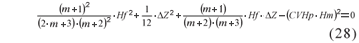in which m is the exponent with respect to discharge in the friction equations; Hm is the mean value of pressure (m) throughout the lateral  calculated by inverting eq. (25); Hf is the total friction loss (m) throughout the lateral; and DZ is the level difference (m), assuming a negative value only in case of downhill slopes.

The following equations must be substituted into eq. (28) prior to its solution:in which L is the length (m) of the lateral  the goal value to be found iteratively; So is the soil slope (%), assumed as uniform; hfe the local head losses (m  equivalent length) at emitter insertions; Khf the friction constant; Se the emitter spacing (m); D the internal lateral diameter (m); n the adjusted empirical coefficient.

Analogously to eq. (27), there is no problem in solving eq. (28) by using either of the most traditional methods (Newton-Raphson, Secant or bisection). The current approach disregards higher order terms of the Christiansen coefficient (F), in which F = 1 / (m + 1), valid for laterals contemplating a large number of emitters (i.e. N > 20). However, the exact length of lateral and consecutively the number of emitters (N) are not known a priori (i.e. L may be very short under high slope situations if at the same time Hm is low and CVq is rigorously low), which then would require the full equation for calculating F regardless of its former simplification.

As N must be a non-decimal number, once a L value is found from eq. (28), N must be truncated (for NR) preferably towards to the nearest smaller integer value in order to obtain a little gain in terms of pressure throughout the lateral, which has in addition a benefit of preventing clogging of emitters due small particles of sediments. As a consequence, a new lateral length (LR) is then recalculated: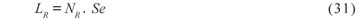Thus, the lateral inlet pressure (Hinl, m) and the pressure related to each i emitter (Hi, m) may be calculated, respectively, according to: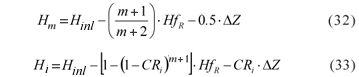where HfR and DZR are the recalculated DZ and Hf, in an analogous way provided by equations (29) and (30), respectively, by replacing LR by L. Likewise eq. (28), DZ is here is also assumed as negative for declivity situations; CRi is the relative length, i.e. the length between the first and the ith emitter, is given by: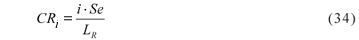Consequently, the discharge (qi, L h1) at each emitter may be obtained by:An equation that accounts for both emitter variation and system pressure variation is one denoting the uniformity of emission, which was modified and redefined for design purposes, as pointed out by Bralts (1986). However, despite the existence of a number of equations to assess the uniformity (i.e. absolute emission uniformity, statistic uniformity etc.), Faveta & Botrel (2001) have compared all these equations and have shown strong correlations among them, which allows the adoption of the one that most suits the user's need. At the design stage, the statistical uniformity (US, %) of water depth can be used (Juana et al., 2004), according to (Wilcox & Swailes, 1947):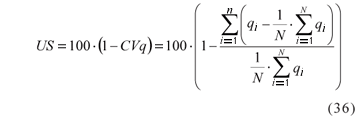There are more factors affecting the uniformity of micro-irrigation than that of sprinkler irrigation besides manufacturer's variation (Wu, 1997). These other factors might be the grouping of emitters, plugging and temperature. The effect of temperature on emitter flow can be neglected when a turbulent flow emitter is used (Peng et al., 1986), whereas plugging of emitters is developed with respect to time and occurs in the form of partial plugging and complete plugging. As partial pluggings are difficult to evaluate, plugging evaluations are taken by using completely plugged emitters (Wu, 1997).

For illustrative purposes values for length were simulated from variations in the input variable CVf (2%, 4%, 6%, 8% and 10%), considering a low-pressure irrigation system designed in a 2% downhill uniform slope (So =  2). The emitter spacing was 1 m (i.e. coffee plantation paddock design) mounted throughout a ½" diameter (or 0.0165 m) lateral and was considered grouped in one per tree; the local head pressure losses due to emitter insertions (hfe) was 0.1 m; the project mean point-source discharge of 4.0 L h1 was considered in principle, given that the emitter equation qi = 1.1134 Hi0.5 was provided (orifice-type emitters working under full turbulent flow, according to x value); and the Blasius constants were assumed (Khf = 0.00078; m = 1.75; n = 4.75). As a project criterion, a relaxed CVq of 10% was considered. The rest of variables were calculated; such as CVHp, lateral, losses by friction, inlet head pressure and uniformity.

With the results of the simulation (Table 1), the user can perform the same simulations by running the following procedure: (i) calculate CVHp from eq. (27); (ii) calculate Hm from eq. (23); iii) calculate L from the combination of equations (28), (29) and (30); (iv) calculate Hinl from eq. (32); (v) calculate Hi from the combination of equations (31), (34), HfR through eq. (30) and eq. (33); (vi) calculate qi (eq. 35); and finally (vii) calculate discharge statistic uniformity of design according to eq. (36).

The required inlet head pressure tend to decrease as CVf increases, and the same is verified in the relationship between Hf and Hinl (Table 1). The statistic uniformity of discharge tends to follow CVf increasing, as CVHp decreases. Interesting to note is that, when CVf = 10%, all results increase or decrease abruptally. From this value on (i.e. CVf > 0.1), there was no convergence for CVHp. This CVf could be considered a critical value, for example, for the scenario assumed (So =  2, D = 0.0165 m etc.).

The better manufacturing of emitters implies a lower CVf value, which allows for lengthening of laterals for a given soil slope, as shown in Figure 1 [the combination of the pairs i vs qi Eq. (35)], however this does not necessarily mean that the discharge statistical uniformity throughout lateral will be more homogenous. It is possible to note that the flatter is the shape of the curve the shorter it is the simulated lateral length. Corroborating to this, a decreasing tendency in statistical uniformity is also highlighted as emitters are more uniformly made and hence resulting in lower CVf values (Figure 2).

As outlined by Wu (1997), the sum of squares relationship shown in the second term of eq. (27) indicates the effect that the hydraulic design will be less significant when the emitters have high manufacturer variations. Hence, the flatter slopes obtained from the higher CVfs in Figure 1, corroborated by Figure 2, reinforce such relationship. Another important characteristic to verify in Figure 1 is not only the distribution of source-point discharge values but also the limits of the corresponding head pressure values (Hi), often leading to quite distant values of qi from the suggested mean discharge (4.0 L h1). The designed mean source-point discharge decreased from 4.36 to 4.15 L h1, as well as for the emitter flow relative variation [(generally called qvar = (qmax  qmin)/qmax], going from 24.48 to 2.91%.

From the lowest (i.e. least variable) to the highest value of CVf, this simple emitter attribute alteration has reduced the lateral length for more than one half, although a 2% CVf still led to an acceptable value of micro-irrigation project uniformity (i.e. greater than 90%). The choice of a 10% CVf would lead to the lowest pressure head at the beginning of the lateral (13.98 m), a value 64% lower than the necessary pressure head if a 2% CVf emitter were selected. Thus, a 10% CVf emitter would result in a more economical system with respect to energy expense considered alone, but would be the shorter lateral line on the other hand. Wu (1997) report that when the manufacturer's variation of selected emitters is selected to be < 10%, which is very easy to achieve, the system uniformity with CV < 20% can be achieved when the qvar is 30%.

Factors such as CVf, CVH, CVq, Hi and x should be carefully considered when a micro-irrigation system is designed and managed in order to ensure an application of water that is as uniform as possible. However, regular evaluation of irrigations should take place when systems are working so that they can be correctly maintained and can closely perform according to their original design (Pereira, 1999). Moreover, one can also create an optimisation model to find the best trade-off between lateral length and CVf, thereby maximising profits; however this is not the primary goal of this work. The costs of different emitters, supposedly varying with the level of manufacturing, need to be compared as well, since it is useful to assess the profitability of an irrigation system by taking into account the fixed costs involved. It is often necessary when dealing with manufacturing variation of a particular emitter to reinforce its applicability in practical situations of systems design. Therefore, it is always worthwhile to outline some crucial concepts and methodologies by bringing them in an accessible manner for educational purposes, i.e. by deducing eq. (27) in order to support the choice of the lowest (or even highest) CVf .

ACKNOWLEDGEMENT

These authors are grateful to the following Brazilian Institutions for their financial support: Federal Department of Science and Technology (MCT), National Scientific and Technological Development Council (CNPq), Sao Paulo State Scientific Foundation (FAPESP) and National Institute of Science and Technology in Irrigation Engineering (INCTEI).

Accepted December 12, 2008

APPENDIX

List of symbols:

q - Source-point discharge [L3 t1]

K - Coefficient in emitter discharge formula [L3-x t1]

x - Exponent of emitter discharge equation

ρ - Correlation

δ2 - Variance

d - Deviation or differential

CVq - Coefficient of variation in discharge

CVH - Coefficient of variation of pressure heads

CVHp - Coefficient of variation of pressure heads at design stage

CVK = CVf - Coefficient of variation in discharge due to manufacture

E(q) - Expectation of q [L3 t1]

L - Lateral length of emitter insertion [L]

Khf - Constant in empirical equation of head loss

m - Exponent of discharge in empirical equation of head loss

n - Exponent of diameter in empirical equation of head loss

N - Number of emitters

Hf - Total head losses along a working lateral [L]

ΔZ - Elevation or level difference [L]

So -Slope [L, if divided by 100; otherwise is dimensionless]

Se - Emitter spacing [L]

hfe - Equivalent length of emitter [L]

D - Lateral internal diameter [L]

F - Christiansen' factor

CR - Relative length

US - Statistic uniformity of discharge

List of subscripts:

i - Order of a number of series

inl - inlet

K - Coefficient in emitter discharge formula

m - Mean

R - Recalculated

x - Exponent of emitter discharge equation

• ANYOJI, H.; WU, I.P. Statistical approach for drip lateral design. Transactions of ASAE, v.30, p.187-192, 1987.
• ASAE. ASAE standards engineering practices data 43 ed. St. Joseph: ASAE, 1996. 864p.
• BRALTS, V.F. Field performance and evaluation. In: NAKAYAMA, F.S.; BUCKS, D.A. (Ed.) Trickle irrigation for crop production Amsterdam: Elsevier, 1986. p.216-240. (Development in Agricultural Engineering, 9).
• BURDEN, R.L.; FAIURES, J.D. Numerical analysis 8 ed. New York: Brooks Cole, 2004. 847p.
• FAVETA, G.M.; BOTREL, T.A. Uniformity of localized irrigation systems: validation of equations. Scientia Agricola, v.58, p.427-430, 2001.
• FRIZZONE, J.A.; VIEIRA, A.T.; PAZ, V.P.S.; BOTREL, T.A. Hydraulic characterisation of a drip tape. Revista Brasileira de Engenharia Agrícola e Ambiental, v.2, p.278-283, 1998.
• JUANA, L.; LOSADA, A.; RODRIGUEZ-SINOBAS, L.; SÁNCHEZ, R. Analytical relationships for designing rectangular drip irrigation units. Journal of Irrigation and Drainage Engineering, v.130, p.47-59, 2004.
• KELLER, J.; BLIESNER, R.D. Sprinkler and trickle irrigation New York: Von Nnostrand Reinhold, 1990. 582p.
• LINDLEY, D.V. Introduction to probability and statistics (Part I). Cambridge: University Press, 1965.
• MOORE, D.S. The basis practice of statistics New York: W. H. Freeman, 1995. 680p.
• PENG, G.F.; WU, I.P.; PHENE, C.J. Temperature effects on drip line hydraulics. Transactions of ASAE, v. 29, p.211-215, 1986.
• PEREIRA, L.S. Higher performance through combined improvements in irrigation methods and scheduling: a discussion. Agricultural Water Management, v.40, p.153-169, 1999.
• WILCOX, J.C.; SWAILES, G.E. Uniformity of water distribution by some undertree orchard sprinklers. Scientific Agricultural, v.27, p.565-583, 1947.
• WU, I.P. An assessment of hydraulic design of micro-irrigation systems. Agricultural Water Management, v.32, p.275-284, 1997.
• *
Corresponding author <

# Publication Dates

• Publication in this collection
04 Aug 2009
• Date of issue
Aug 2009

• Accepted
12 Dec 2008